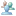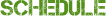# Course: Geometry And Discrete Mathematics

Geometry And Discrete Mathematics
Program ID: MATH
Course ID : MGA4U
Course Cost:980.00
Course Hours:110

This course enables students to broaden mathematical knowledge and skills related to abstract mathematical topics and to the solving of complex problems. Students will solve problems involving geometric and Cartesian vectors, and intersections of lines and planes in three-space. They will also develop an understanding of proof, using deductive, algebraic, vector, and indirect methods. Students will solve problems involving counting techniques and prove results using mathematical induction.

none

##### Learning Outcomes and Career Opportunities
High School Credit